# Borrow and Carry Bit for Subtraction

Similar to the usage of the carry bit when adding there are mechanisms for subtracting that allow to integrate the result of subtraction of the lower bits into the subtraction of the next higher block of bits, where necessary.

There are two ways to do this, that are trivially equivalent by a simple not operation:

• borrow bit (also borrow flag)
• carry bit (also carry flag)

Often CPUs use what is the carry bit for addition and interpret it as borrow bit for subtraction.

Here are the two ways:

## Borrow Bit

It is assumed, that the CPU-word is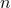bits long, so calculations are performed modulo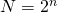. Further it is assumed, that the rangeis preferred, so all sign issues are excluded for the moment.

When subtracting two numbers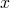,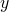from each other like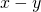and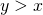, the provided result is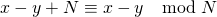and the borrow bit is set (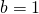), to indicate that the subtraction caused „underflow“, which had to be corrected by addedin order to get into the desired range.

In the „normal“ case where, the provided result is simply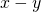and the borrow bit is not set ().

The next round of subtraction takes the borrow bit into account and calculates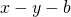, where the condition becomesand the result is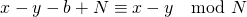or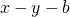respectively. This is how some of the older readers used to do it in school on paper, but of course with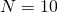.

Now the typical integer arithmetic of current CPUs uses Two’s complement, which means that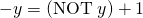. Combining this with the previous results in calculating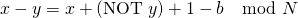At this point some CPU-designers found it more natural, to use the carry bit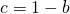instead of the borrow bit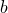.

## Carry Bit

When subtracting two numbers,from each other likeand we have, the provided result isand the carry bit is not set (), to indicate that the subtraction caused „underflow“, which had to be corrected by addedin order to get into the desired range.

In the „normal“ case where, the provided result is simplyand the carry bit is set ().

The next round of subtraction takes the borrow bit into account and calculates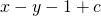, where the condition becomes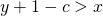and the result is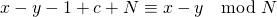orrespectively.

Now two’s complement withthis can be written asor with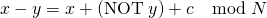These two ways are really equivalent and easily transformed into each other. Neither of them provides big advantages, apart from the fact that we have the unnecessary confusion, because it depends on the CPU design, which of the two variants is used.

## Recovery of Borrow or Carry bit

The borrow bit is calculated and used during subtractions that are done at assembly language level, but higher languages like C or Java do provide access to this information. It is relatively easy to recover the carry bit in the case of addition based on,and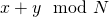.

This possible as well for the subtraction. Quite easily the comparison betweenandor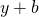could be done before the subtraction. This would work, but it is kind of inefficient, because under the hood the comparison is just a subtraction with discarding the result and keeping the flags. So the subtraction is performed twice. This might not be such a bad idea, because a compiler could recognize it or the work of subtracting twice could be neglectable compared to the logic for an „optimized“ recovery, based on some logic expression of certain bits from,and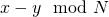.